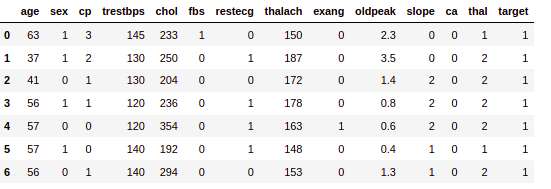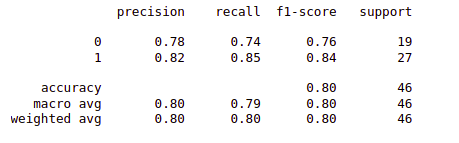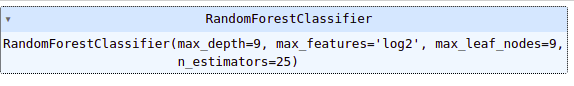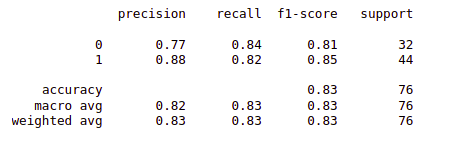GeeksforGeeks App
Open AppBrowser
Continue

# Random Forest Hyperparameter Tuning in Python

In this article, we shall implement Random Forest Hyperparameter Tuning in Python using Sci-kit Library.

Sci-kit aka Sklearn is a Machine Learning library that supports many Machine Learning Algorithms, Pre-processing Techniques, Performance Evaluation metrics, and many other algorithms. Ensemble Techniques are considered to give a good accuracy score among all the Machine Learning Algorithms. Since Machine Learning are classified into Supervised and Unsupervised Learning, we have two types of approach in Supervised Learning that is Regression and classification. Talking of Classification, let us consider one of the ensembles technique i.e., the Random Forest algorithm. While building a Classification model, we always think about what value should be assigned to the Hyperparameters. Hyperparameters are similar to parameters but the only difference is there is no one specific value to these Hyperparameters.

Since we are talking about Random Forest Hyperparameters, let us see what different Hyperparameters can be Tuned.

## Random Forest Hyperparameters

#### 1. n_estimators

Random Forest is nothing but a set of trees. It is an extended version of the Decision Tree in a very optimized way. One issue here might arise is how many trees need to be created. n_estimator is the hyperparameter that defines the number of trees to be used in the model. The tree can also be understood as the sub-divisions.

`By default: n_estimators=100`

#### 2. max_features

In order to train the Machine learning model, the given dataset should contain multiple features/variables to predict the label/target. Max_features limits a count to select the maximum features in each tree.

`By default: max_features="sqrt" [available: ["sqrt", "log2", None}]`

#### 3. max_depth

A tree is incomplete without a split or child node. max_depth determines the maximum number of splits each tree can take. If the max_depth is too low, the model will be trained less and have a high bias, leading the model to underfit. In the same way, if the max_depth is high, the model learns too much and leads to high variance, leading the model to overfit.

`By default: max_depth=None`

#### 4. max_leaf_nodes

We have a tree and know what max_depth is used for. Talking of a Tree, each tree is used to split into multiple nodes. But how many divisions of nodes should be done is specified by max_lead_nodes. max_leaf_nodes restricts the growth of each tree.

`By default: max_leaf_nodes = None;  (takes an unlimited number of nodes)`

#### 5. max_sample

Apart from the features, we have a large set of training datasets. max_sample determines how much of the dataset is given to each individual tree.

`By default: max_sample = None; (this means data.shape is taken)`

#### 6. min_sample_split

Since ensemble algorithms are weak learners and are derived from strong learners, Random Forest which is a Weak Learner depends on Decision Tree decisions.  min_sample_split determines the minimum number of decision tree observations in any given node in order to split.

`By default: min_sample_split = 2 (this means every node has 2 subnodes)`

For a more detailed article, you can check this:  Hyperparameters of Random Forest Classifier

## Random Forest Hyperparameter Tuning in Python using Sklearn

Sklearn supports Hyperparameter Tuning algorithms that help to fine-tune the Machine learning models. In this article, we shall use two different Hyperparameter Tuning i.e., GridSearchCV and RandomizedSearchCV.

Import the required modules that are needed to fine-tune the Hyperparameters in Random Forest.

## Python3

 `from` `sklearn.metrics ``import` `classification_report``from` `sklearn.model_selection ``import` `train_test_split``import` `pandas as pd``from` `sklearn.ensemble ``import` `RandomForestClassifier``from` `sklearn.model_selection ``import` `GridSearchCV,\``RandomizedSearchCV`

## Python3

 `data ``=` `pd.read_csv(``    ``"https://raw.githubusercontent.com/lucifertrj/"``    ``"100DaysOfML/main/Day14%3A%20Logistic_Regression"``    ``"_Metric_and_practice/heart_disease.csv"``)``data.head(``7``)`

Output:First five rows of the dataset

## Python3

 `data[``'target'``].value_counts()`

Output:

```1    165
0    138
Name: target, dtype: int64```

### Prepare and Split the Data

We shall consider all the columns as our input i.e., features. And the target column will act as the label that we need to predict. Using train_test_split we split out the test size and train size by 25:75 percent.

## Python3

 `X ``=` `data.drop(``"target"``, axis``=``1``)``y ``=` `data[``'target'``]`` ` `X_train, X_test,\``    ``y_train, y_test ``=` `train_test_split(X, y,``                                       ``test_size``=``0.25``,``                                       ``random_state``=``42``)``X_train.shape, X_test.shape`

Output:

`((227, 13), (76, 13))`

### Build Random Forest Model

First, we will train the model with default Random Forest Classifier Hyperparameters.

## Python3

 `model ``=` `RandomForestClassifier()``model.fit(X_train, y_train)`` ` `# predict the mode``y_pred ``=` `model.predict(X_test)`` ` `# performance evaluatio metrics``print``(classification_report(y_pred, y_test))`

Output:Classification report for the Random Forest Model

## Python3

 `param_grid ``=` `{``    ``'n_estimators'``: [``25``, ``50``, ``100``, ``150``],``    ``'max_features'``: [``'sqrt'``, ``'log2'``, ``None``],``    ``'max_depth'``: [``3``, ``6``, ``9``],``    ``'max_leaf_nodes'``: [``3``, ``6``, ``9``],``}`

Note: Hyperparameter Tuning doesn’t work all the time. Sometimes the Default Hyperparameters are also considered to be the best estimators.

### Hyperparameter Tuning- GridSearchCV

First, let’s use GridSearchCV to obtain the best parameters for the model. For that, we will pass RandomFoestClassifier() instance to the model and then fit the GridSearchCV using the training data to find the best parameters.

## Python3

 `grid_search ``=` `GridSearchCV(RandomForestClassifier(),``                           ``param_grid``=``param_grid)``grid_search.fit(X_train, y_train)``print``(grid_search.best_estimator_)`

Output:Parameters obtained by using GridSearchCV

#### Update the Model

Now we will update the parameters of the model by those which are obtained by using GridSearchCV.

## Python3

 `model_grid ``=` `RandomForestClassifier(max_depth``=``9``,``                                    ``max_features``=``"log2"``,``                                    ``max_leaf_nodes``=``9``,``                                    ``n_estimators``=``25``)``model_grid.fit(X_train, y_train)``y_pred_grid ``=` `model.predict(X_test)``print``(classification_report(y_pred_grid, y_test))`

Output:Classification report for the Random Forest Model

### Hyperparameter Tuning- RandomizedSearchCV

Now let’s use RandomizedSearchCV to obtain the best parameters for the model. For that, we will pass RandomFoestClassifier() instance to the model and then fit the RandomizedSearchCV using the training data to find the best parameters.

## Python3

 `random_search ``=` `RandomizedSearchCV(RandomForestClassifier(),``                                   ``param_grid)``random_search.fit(X_train, y_train)``print``(random_search.best_estimator_)`

Output:Parameters obtained by using RandomizedSearchCV

#### Update the model

Now we will update the parameters of the model by those which are obtained by using RandomizedSearchCV.

## Python3

 `model_random ``=` `RandomForestClassifier(max_depth``=``3``,``                                      ``max_features``=``'log2'``,``                                      ``max_leaf_nodes``=``6``,``                                      ``n_estimators``=``100``)``model_random.fit(X_train, y_train)``y_pred_rand ``=` `model.predict(X_test)``print``(classification_report(y_pred_rand, y_test))`

Output:Classification report for the Random Forest Model

My Personal Notes arrow_drop_up